GreenBuildings2

# GreenBuildings 2: Imputing Missing Values With Scikit-Learn¶

1. Introduction

2. Analyzing Distributions & Correlations

3. Imputing Missing Values With Scikit-Learn

4. Next Steps

## Introduction ¶

This is the second post in a series of blog posts about building a predictive model of green house gas emissions of buildings in NYC. In my first post I covered how to perform

• Exploratory data analysis
• Identify and remove outliers

In this current blog post I will cover the very important topic of

• Imputing missing values

Specifically I will cover imputations techniques#Regression) using Scikit-Learn's impute module using both point estimates (i.e. mean, median) using the SimpleImputer class as well as more complicated regression models (i.e. KNN) using the IterativeImputer class. The later requires that the features in the model are correlated. This is indeed the case for our dataset and in our particular case we also need to transform) the feautres in order to discern a more meaningful and predictive relationship between them. As we will see, the transformation of the features also gives us much better results for imputing missing values.

I should remark that one must always first determine if missing values are missing at random or missing not at random. Values that are missing not at random are dangerous to impute. See here for more discussion. In this case we don't really know why the data is missing and will impute the missing values. Imputatation of missing values will introduces bias into our dataset, but as we saw in the last post that nearly 50% of the data had missing values, so we have to do something.

Lastly we remark that the use of KNN, while flexible and works quite well is not the best model imputing missing values for models in production. This is because KNN is a memory-based model and stores the entire dataset which is impracticle for large datasets and models which require low latency. It was used in this case in-lieu of more traditional regression models because the features whose values we wished to impute did not follow a normal distribution.

## Analyzing Distributions & Correlations ¶

We first import the necessary packages. In the previous post I talked about using Google Cloud Platform and won't go over the details of the configuations, but declare them in my list of import below:

In :
import numpy as np
import pandas as pd
import matplotlib.pyplot as plt
import seaborn as sns
%matplotlib inline
sns.set()

import warnings
warnings.filterwarnings('ignore')

import json
import pandas_gbq

credentials = service_account.Credentials\
.from_service_account_file('./derby.json')

client = bigquery.Client(project     = credentials.project_id,
credentials = credentials)

pandas_gbq.context.credentials = credentials
pandas_gbq.context.project     = credentials.project_id


Let's first get the names of the columns (I actually forgot what they were):

In :
result = client.query("""
SELECT
column_name
FROM
db_gb.INFORMATION_SCHEMA.COLUMNS
WHERE
table_name = 'no_outlier_data'
""")

for row in result.result():
print(row)

Energy_Star
Site_EUI
NGI
EI
WI
GHGI
OPSFT
Age
Residential


Now that I know the names of the columns let's see exactly what the number of null values each column has and graph these counts. To make it more interesting I break this down even further by incorporating whether the building was Multi-family or Office space:

In :
query = """
SELECT
Residential,
SUM(CASE WHEN Energy_Star IS NULL THEN 1 ELSE 0 END)  AS Energy_Star_NA,
SUM(CASE WHEN Site_EUI IS NULL THEN 1 ELSE 0 END)  AS Site_EUI_NA,
SUM(CASE WHEN NGI IS NULL THEN 1 ELSE 0 END)  AS NGI_NA,
SUM(CASE WHEN WI IS NULL THEN 1 ELSE 0 END)  AS WI_NA,
SUM(CASE WHEN EI IS NULL THEN 1 ELSE 0 END)  AS EI_NA,
SUM(CASE WHEN GHGI IS NULL THEN 1 ELSE 0 END) AS GHGI_NA,
FROM
db_gb.raw_data
GROUP BY
Residential
"""

# reshape the dataframe
num_nulls_df2 = num_nulls_df.melt(id_vars='Residential')\
.rename(columns={"value":"Count"})

# bar plot with break outs on residential column
plt.figure(figsize=(10,3))
sns.barplot(data=num_nulls_df2,
x='variable',
y='Count',
hue='Residential')

Downloading: 100%|██████████| 2/2 [00:00<00:00,  5.44rows/s]

Out:
<matplotlib.axes._subplots.AxesSubplot at 0x7fcea4a389b0>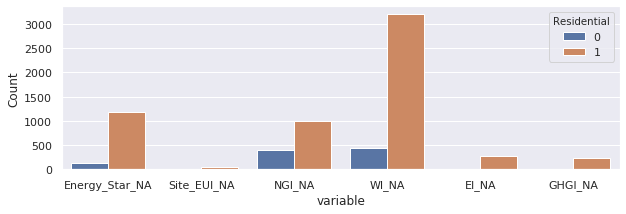We can see generally residential buildings have more missing values than office buildings. This isn't quite a fair comparison as the number of residential buildings could be much larger than the number of office buildings and therefore inflating the number of missing values.

What we are really interested in is the percentage of missing values in each column and within each building type:

In :
query = """
SELECT
Residential,
SUM(CASE WHEN Energy_Star IS NULL THEN 1 ELSE 0 END) / COUNT(*) AS Energy_Star_NA,
SUM(CASE WHEN Site_EUI IS NULL THEN 1 ELSE 0 END) / COUNT(*) AS Site_EUI_NA,
SUM(CASE WHEN NGI IS NULL THEN 1 ELSE 0 END) / COUNT(*) AS NGI_NA,
SUM(CASE WHEN WI IS NULL THEN 1 ELSE 0 END) / COUNT(*) AS WI_NA,
SUM(CASE WHEN EI IS NULL THEN 1 ELSE 0 END) / COUNT(*) AS EI_NA,
SUM(CASE WHEN GHGI IS NULL THEN 1 ELSE 0 END) / COUNT(*) AS GHGI_NA,
FROM
db_gb.no_outlier_data
GROUP BY
Residential
"""

# reshape the dataframe
frac_nulls_df2 = frac_nulls_df.melt(id_vars='Residential')\
.rename(columns={"value":"Fraction Null"})
# bar plot with break outs on residential column
plt.figure(figsize=(10,3))
sns.barplot(data=frac_nulls_df2,
x='variable',
y='Fraction Null',
hue='Residential')

Downloading: 100%|██████████| 2/2 [00:00<00:00,  5.71rows/s]

Out:
<matplotlib.axes._subplots.AxesSubplot at 0x7fce9f4f7390>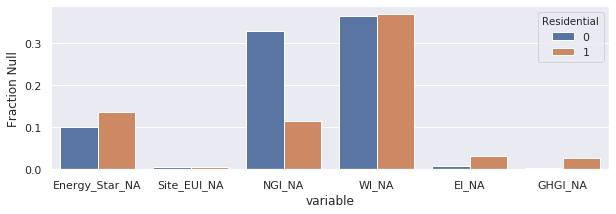This is a much more meaningful comparison! Note that we are using the data without any outliers, that is the table resulting from the last post. We can see that,

• Water intensity (WI) is the field with highest percentage of missing values

• Natural Gas Intensity (NGI) has the second highest percentage of missing values.

• Energy_Star also has a sizable amount of missing values.

Site Energy Use Intensity (EUI), Electricity Intensity (EI) and Green House Gas Intensity (GHGI) have negligable amounts of missing values and those records with missing values will be dropped. Note that the sizable difference between Residential values means we have to take building type into account when imputing these values.

Let's pull all the columns to determine the relationship of each feature not only with GHGI (remember the final objective of these posts is to make a model that predicts GHGI), but also with all the other features. We determine the relationships using a heat map of the correlation matrix as we did in the last post:

In :
df = pandas_gbq.read_gbq("""
SELECT
Energy_Star,
Site_EUI,
NGI,
EI,
WI,
GHGI,
Age,
OPSFT,
Residential,
FROM
db_gb.no_outlier_data
""")

Downloading: 100%|██████████| 9834/9834 [00:01<00:00, 5066.82rows/s]

In :
from sklearn.preprocessing import StandardScaler
scaler1 = StandardScaler()
Xs      = scaler1.fit_transform(df)
xs_df   = pd.DataFrame(Xs, columns=df.columns)

fig, ax = plt.subplots(figsize=(8,6))
sns.color_palette("BuGn_r",)
sns.heatmap(xs_df.corr(),
linewidths=.5,
cmap="RdBu_r")

Out:
<matplotlib.axes._subplots.AxesSubplot at 0x7fce8fe4d9b0>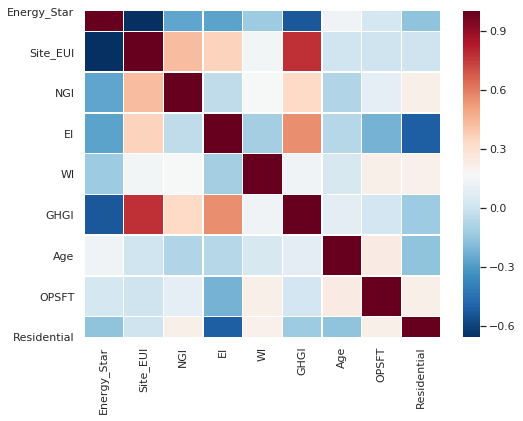We can see that,

• Occupancy Per Square Foot (OPSFT) and Age are not very correlated with GHGI. Therefore we will not concern ourselves with them in our model.

• Water intensity (WI) is not very correlated and has as mentioned has A LOT missing values.

• EI, NGI, Energy_Star, and GHGI, are all highly correlated.

Let's look at the scatter plots of features and GHGI:

In :
sns.pairplot(df,
vars=["Energy_Star","Site_EUI","NGI","EI","GHGI", "WI"],
hue='Residential')

Out:
<seaborn.axisgrid.PairGrid at 0x7fce9f478828>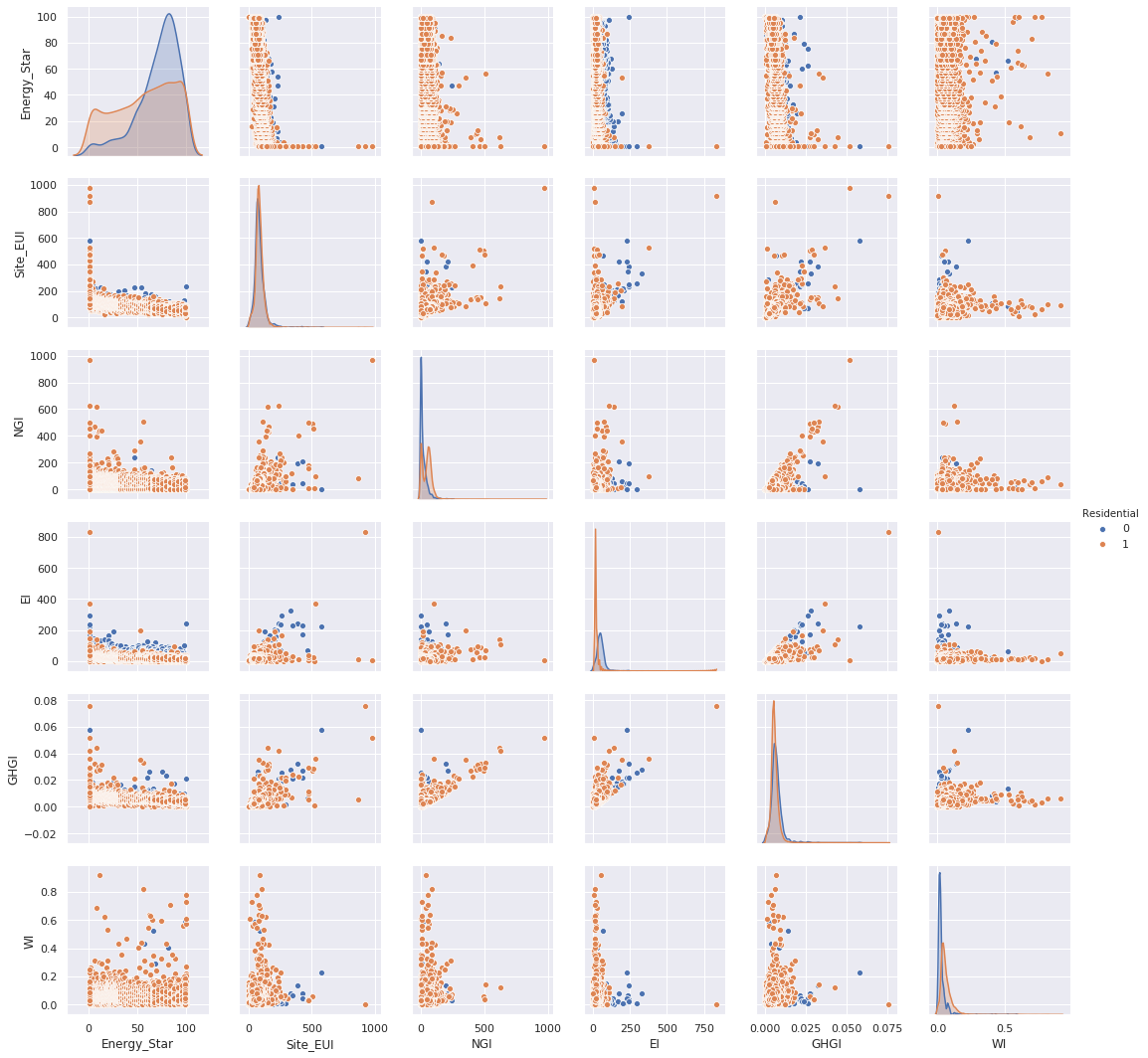In the above graph the off-diagonal grids are the relationship between the variables while the graphs along the diagonal are the density of the variables. We can see from the off-diagonal graphs there is heteroscedasticity between the features and GHGI, however, we won't address this issue too much in this post. The right most column shows that WI is not correlated with GHGI or any of the other features. We therefore will not be imputing missing values for water intensity (WI) and drop it as a feature for the model of GHGI and instead be focusing on imputing missing values for

1. Energy_Star
2. NGI

From the graphs along the diagonal all variables except for Energy_Star have sharply peaked modes) with long tails. Interestingly, NGI and EI seem to be bimodal distributions which will make things more difficult for imputing their missing values. In order to make the relations between features more visible I tried tranforming the data. The first transformation I used was too use the square root of all the variables except for Energy_Star. The reason I chose I the squart root is because it will reduce the sharp-peakedness of the distributions and hopefully make them more normally distributed:

In :
df_sqrt = pandas_gbq.read_gbq("""
SELECT
Energy_Star,
SQRT(Site_EUI) AS Site_EUI,
SQRT(NGI)      AS NGI,
SQRT(EI)       AS EI,
SQRT(GHGI)     AS GHGI,
Residential,
FROM
db_gb.no_outlier_data
""")

sns.pairplot(df_sqrt,
vars=["Energy_Star","Site_EUI","NGI","EI","GHGI"],
hue='Residential')

Downloading: 100%|██████████| 9834/9834 [00:01<00:00, 6043.71rows/s]

Out:
<seaborn.axisgrid.PairGrid at 0x7fce9df10d30>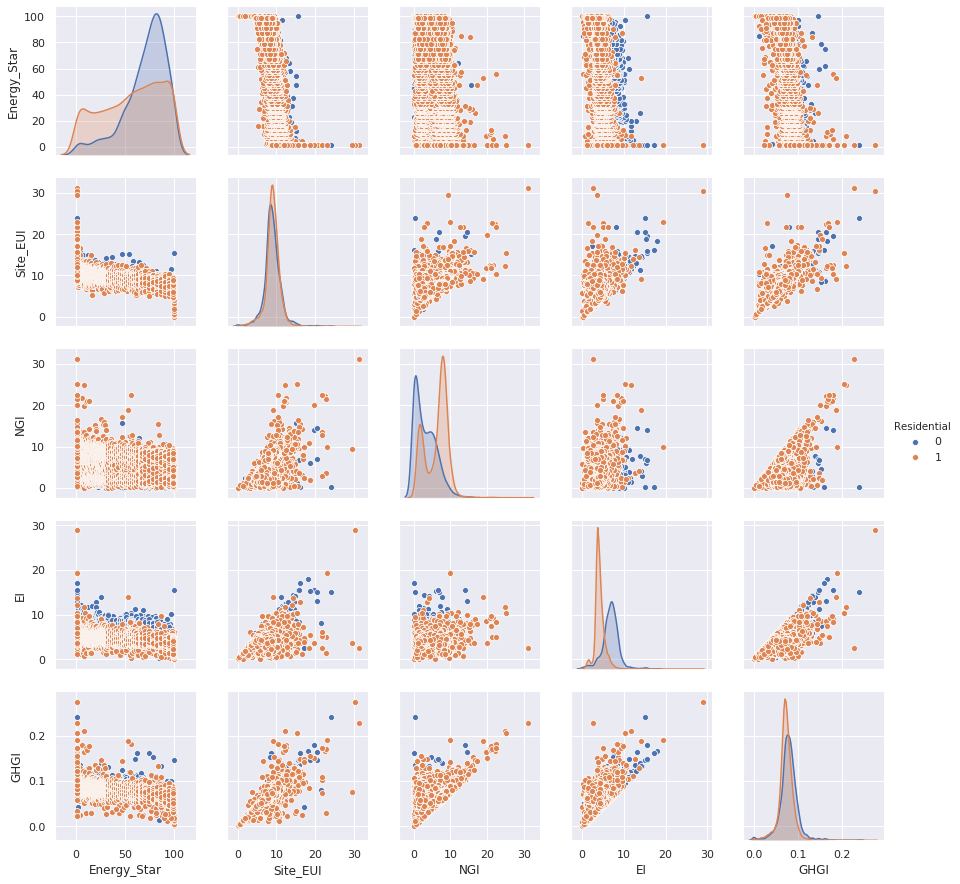We can see that neither Energy_Star nor NGI are normally distributed, meaning that a linear regression model would not be appropriate in this situation and hence choose a more flexible regression model. Interesting the relationship between Energy_Star and Site_EUI seams to be somewhat sigmodal in shape signfiying a non-linear relationship between the two.

The relationship between NGI, Site_EUI and GHGI seems to be linear, but suffering from heteroscedasticity. One way to reduce the variance is to use use log tranformations on the variable. For the purposes of imputing missing values in NGI we only really care heteroscedasticity in the NGI and therefore only transform it. Inorder to avoid the issue of introducing infinities when NGI is zero into we add a 1 when log transforming it:

In :
df_sqrt["LOG_NGI"] = np.log(1 + df_sqrt["NGI"])


Now lets look at the relationship of this variable with respect to the square root of the other features:

In :
sns.pairplot(df_sqrt,
x_vars=['EI','Site_EUI','Energy_Star','GHGI'],
y_vars='LOG_NGI',
hue='Residential',
kind = 'reg',
size=5,
dropna=True)

Out:
<seaborn.axisgrid.PairGrid at 0x7fce9cf25470>